# MP Board Class 10th Science Solutions Chapter 1 Chemical Reactions and Equations

In this article, we will share MP Board Class 10th Science Solutions Chapter 1 Chemical Reactions and Equations Pdf, These solutions are solved subject experts from the latest edition books.

## MP Board Class 10th Science Solutions Chapter 1 Chemical Reactions and Equations

### MP Board Class 10th Science Chapter 1 Intext Questions

Mp Board Class 10 Science Chapter 1 Question 1.
Why should a magnesium ribbon be cleaned before burning in air?
Magnesium metal is highly reactive. In stored conditions, it reacts with oxygen to form magnesium oxide over its outer layer. To remove this layer and to expose the underlying metal into air, the magnesium ribbon is cleaned by sandpaper.

Mp Board 10th Science Chapter 1 Question 2.
Write the balanced equation for the following chemical reactions:

1. Hydrogen + Chlorine → Hydrogen Chloride.
2. Barium Chloride + Aluminium Sulphate → Barium Sulphate + Aluminium Chloride.
3. Sodium + Water Sodium Hydroxide + Hydrogen.

Balanced equations for the chemical reactions are as follows:

1. H2(g) + Cl2(g) → 2HCl(g)
2. 3BaCl2(aq) + Al2(SO4)3(aq) → 3BaSO4(s) + 2AlCl3(aq)
3. 2Na(s) + 2H2O(l) → 2NaOH(aq) + H2(g)

Mp Board Class 10th Science Chapter 1 Question 3.
Write a balanced chemical equation with state symbols for the following reactions:

1. Solutions of barium chloride and sodium sulphate in water react to give insoluble barium sulphate and the solution of sodium chloride.
2. Sodium hydroxide solution (in water) reacts with a hydrochloric acid solution (in water) to produce sodium chloride solution and water.

Balanced chemical equations with state symbols for the required reactions are as follows:

1. BaCl2(aq) + Na2SO4(aq) → BaSO4(s) + 2NaCl(aq)
2. NaOH(aq) + HCl(aq) + NaCl(aq) + H2O(l)

Intext Questions Page No. 10

Class 10 Science Chapter 1 Mp Board Question 1.
A solution of a substance ‘X’s used for white-washing.
(i) Name the substance ‘X’ and write its formula.
(ii) Write the reaction of the substance ‘X’ named in (i) above with water.
(i) ‘X’ is calcium oxide. It is used in white-washing and its chemical formula is CaO.
(ii) Calcium oxide reacts vigorously with water to form calcium hydroxide (slaked lime).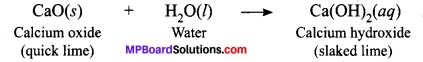Mp Board Class 10 Science Solution English Medium Question 2.
Why is the amount of gas collected in one of the test tubes in double of the amount collected in the other? Name this gas.
Water (H2O) contains two parts of hydrogen and one part of oxygen. The ratio of water components i.e., hydrogen and oxygen is 2:1. Therefore, the amount of hydrogen and oxygen produced after water electrolysis is in a ratio of 2:1. This is why during electrolysis the amount of gas collected in hydrogen’s test tubes is double the amount collected in the oxygen’s test tube.

1. Take a plastic mug. Drill two holes at its base and fit rubber stoppers in these holes. Insert carbon electrodes in these rubber stoppers as shown in Figure.
2. Connect these electrodes to a 6-volt battery.
3. Fill the mug with water such that the electrodes are immersed. Add a few drops of dilute sulphuric acid to the water.
4. Take two test tubes filled with water and invert them over the two carbon electrodes.
5. Switch on the current and leave the apparatus undisturbed for some time.
6. You will observe the formation of bubbles at both electrodes. These bubbles displace water in the test tubes.
7. Is the volume of the gas collected the same in both the test tubes?
8. Once the test tubes are filled with the respective gases. Remove them carefully.
9. Test these gases one by one by bringing a burning candle close to the mouth of the test tubes.

Caution:

1. This step must be performed carefully by the teacher.
2. What happens in each case?
3. Which gas is present in each test tube?

Science Class 10 Chapter 1 Mp Board Observations:
On electrolysis of water, hydrogen and oxygen gas is produced. Hydrogen gas has twice the volume of oxygen gas. Oxygen gas let the burning continue while hydrogen gas burns with a pop sound. The gas collected at the cathode is oxygen while gas collected at the anode is hydrogen.

Intext Questions Page No. 13

Class 10th Science Chapter 1 Mp Board Question 1.
Why does the colour of copper sulphate solution change when an iron nail is dipped in it?
The colour of copper sulphate solution changes when an iron nail is dipped in it because iron is highly reactive than copper. Therefore Iron displaces copper from copper sulphate.

Mp Board Class 10 Science Solution Chapter 1 Question 2.
Give an example of a double displacement reaction other than the one.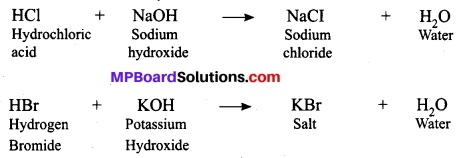Above two reactions represents double displacement reaction. As both electropositive ions of two reactants exchange their electronegative ions and form two new and more stable products, show two replacements at a time and thus called double displacement reactions. In these reactions, acid and base exchange ions to form two new compounds. Hence, it is a double displacement reaction.

Class 10 Science Mp Board Chapter 1 Question 3.
Identify the substances that are oxidized and the substances that are reduced in the following reactions.

1. Na(s) + O2(g) → 2NaO(s)
2. CuO(s) + H2(g) → Cu(s) + H2O(l)

1. Sodium (Na) is oxidized as it gains oxygen and oxygen gets reduced.
2. Copper oxide (CuO) is reduced to copper (Cu) while hydrogen (H) gets oxidised to water (H2O).

### MP Board Class 10th Science Chapter 1 NCERT Textbook Exercises

Mp Board Solution Class 10 Science Chapter 1 Question 1.
Which of the statements about the reaction below are incorrect?
2PbO(s) + C(s) → 2Pb(s) + CO2(g)
(b) Carbon dioxide is getting oxidised.
(c) Carbon is getting oxidised.
(d) Lead oxide is getting reduced.
(i) (a) and (b)
(ii) (a) and (c)
(iii) (a), (b) and (c)
(iv) all
(i) (a) and (b)
Lead is getting reduced. & Carbon dioxide is getting oxidised.

Class 10th Science Chapter 1 Solutions Question 2.
Fe2O3 + 2Al → Al2O3 + 2Fe. The given reaction is an example of a:
(a) Combination reaction.
(b) Double displacement reaction.
(c) Decomposition reaction.
(d) Displacement reaction.
(d) The reaction is an example of a displacement reaction.

Science Class 10 Mp Board Solutions Question 3.
What happens when dilute hydrochloric acid is added to iron filings? Tick the correct answer.
(a) Hydrogen gas and iron chloride are produced.
(b) Chlorine gas and iron hydroxide are produced.
(c) No reaction takes place.
(d) Iron salt and water are produced.
(a) Hydrogen gas and iron chloride are formed.

Class 10 Science Chapter 1 Solution Question 4.
What is a balanced chemical equation? Why should chemical equations be balanced?
A balanced chemical equation is an equation having an equal number of atoms on both sides (reactant and product side) of the reaction. According to, “the law of conservation of mass”, mass can neither be created nor it can be destroyed. So, in a chemical reaction, the total mass of reactants should be equal to the total mass of the products formed.

Class 10 Mp Board Science Chapter 1 Question 5.
Translate the following statements into chemical equations and then balance them.
(a) Hydrogen gas combines with nitrogen to form ammonia.
(b) Hydrogen sulphide gas burns in air to give water and sulphur dioxide.
(c) Barium chloride reacts with aluminium sulphate to give aluminium chloride and a precipitate of barium sulphate.
(d) Potassium metal reacts with water to give potassium hydroxide and hydrogen gas.
(a) 3H2(g) + N2(g) → 2NH3(g).
(b) 2H2S(g) + 3O2(g) → 2H2O(l) + 2SO2(g).
(c) 3BaCl2(aq) + Al2(SO4)3(aq) → 2AlCl3(aq) + 3BaSO4(s).
(d) 2K(s) + 2H2O(l) → 2KOH(aq) + H2(g).

Mp Board Class 10 Science Book Solution Question 6.
Balance the following chemical equations:
(a) HNO3 + Ca(OH)2 → Ca(NO3)2 +H2O.
(b) NaOH + H2SO4 → Na2SO4 + H2O.
(c) NaCl + AgNO3 → AgCl + NaNO3
(d) BaCl2 + H2SO4 → BaSO4 + HCl.
(a) 2HNO3 + Ca(OH)2 → Ca(NO3)2 + 2H2O.
(b) 2NaOH + H2SO4 → Na2SO4 + 2H2O.
(c) NaCl + AgNO3 → AgCl + NaNO3.
(d) BaCl2 + H2SO4 → BaSO4 + 2HCl.

Class 10 Science Chapter 1 Question Answer In Hindi Question 7.
Write the balanced chemical equations for the following reactions.
(a) Calcium hydroxide + Carbon dioxide → Calcium carbonate + Water.
(b) Zinc + Silver nitrate → Zinc nitrate + Silver.
(c) Aluminium + Copper chloride → Aluminium chloride + Copper.
(d) Barium chloride + Potassium sulphate → Barium sulphate + Potassium chloride.
(a) Ca(OH)2 + CO2 → CaCO3 + H2O.
(b) Zn + 2AgNO3 → Zn(NO3)2 + 2Ag.
(c) 2Al + 3CuCl2 → 2AlCl3 + 3Cu.
(d) BaCl2 + K2SO4 → BaSO4 + 2KCl.

Mp Board Class 10 Science Solution Question 8.
Write the balanced chemical equation for the following and identify the type of reaction in each case.
(a) Potassium bromide (aq) + Barium iodide (aq) → Potassium iodide(aq) + Barium bromide (s).
(b) Zinc carbonate (s) → Zinc oxide (s) + Carbon dioxide (g).
(c) Hydrogen (g) + Chlorine (g) → Hydrogen chloride (g).
(d) Magnesium (s) + Hydrochloric acid (aq) → Magnesium chloride (aq) + Hydrogen (g).
(a) 2KBr (aq) + BaI2 (aq) → 2KI (aq) + BaBr2 (s); Double displacement reaction.
(b) ZnCO3(s) → ZnO(s) + CO2(g); Decomposition reaction.
(c) H2(g) + Cl2(g) → 2HCl(g); Combination reaction.
(d) Mg(s) + 2HCl(aq) → MgCl2(aq) + H2(g); Displacement reaction.

Class 10 Science Chapter 1 Activity 1.2 Question 9.
What does one mean by exothermic and endothermic reactions? Give examples.
Exothermic reactions:
Reactions in which energy is evolved are called exothermic reactions.
Chemicalreactionsmayreleaseenergyintheformofheat, light or sound.

Examples: Decomposition of glucose, the formation of hydroxides:
Mg + 2H2O → Fe Mg(OH)2 + H2 + Heat
C + O2 → CO2 + Heat
N2 + 3H2 → 2NH3 + Heat

Reactions in which energy is absorbed are known as endothermic reactions.
These kinds of reactions require energy in order to proceed.
For example: In photosynthesis, plants take photo-energy from the sun to convert carbon dioxide and water into glucose and oxygen,
N2 + O2 → 2NO + Heat
H2SO4(g) → SO3(g) + H2O(g) + Heat
C + 2S → CS2 + Heat

Class 10 Science Chapter 1 Question 10.
Why is respiration considered an exothermic reaction? Explain.
During respiration at the cellular level, food is decomposed to simpler substances releasing carbon dioxide (that we exhale) and water with a release of huge amount of energy. Hence, it is called exothermic reaction. Glucose combines with oxygen in the cells and provides energy.
C6H12O6(aq) + 6O2(g) → 6CO2(g) + 6H2O (l) + Energy.
Since a large amount of energy is released, it is an exothermic reaction.

Mp Board Solution Science Class 10th Science Question 11.
Why are decomposition reactions called the opposite of combination reactions? Write equations for these reactions.
Decomposition reactions involve breaking down of compounds to form two or more substances. These reactions require energy to proceed. Thus, they are the exact opposite of combination reactions in which two or more substances combine to give a new substance.
Examples:

1. ZnCO3(s) → ZnO(s) + CO2(g); Decomposition reaction.
2. H2(g) + Cl2(g) → 2HCl(g); Combination reaction.

In the first equation, since ZnCO3 is broken down into ZnO and CO2 it is a decomposition reaction. In the second equation, H2 and Cl2 combine to give a new substance HCl. Therefore, it is a combination reaction.

Double Displacement Reaction Class 10 Question 12.
Write one equation each for decomposition reactions where energy is supplied in the form of heat, light or electricity.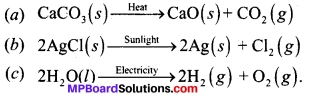Class 10 Science Chapter 1 Solutions Question 13.
What is the difference between displacement and double displacement reactions? Write equations for these reactions.
In a displacement reaction, a more reactive element displaces a less reactive element from a compound.
A + BX → AX + B; where A is more reactive than B.
In a double displacement reaction, two atoms or a group of atoms switch places to form new compounds.
AB + CD → AD + CB
For example:
Displacement reaction:
CuSO4(aq) + Zn(s) → ZnSO4(aq) + Cu(s)

Double displacement reaction:
Na2SO4(aq) + BaCl2 (aq) → BaSO4(s) + 2NaCl(aq)

Question 14.
In the refining of silver, the recovery of silver from silver nitrate solution involved displacement by copper metal. Write down the reaction involved.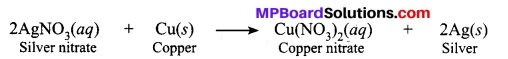Question 15.
What do you mean by a precipitation reaction? Explain by giving examples.
When a compound of product settles down as precipitate at the end of the reaction, it is called a precipitation reaction. In this type of reaction, an ‘insoluble solid called precipitate is formed.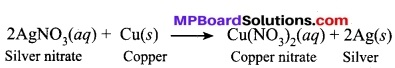In this reaction, silver is obtained as a precipitate. Hence, it is a precipitation reaction.

Question 16.
Explain the following in terms of gain or loss of oxygen with two examples each?
(a) Oxidation
(b) Reduction
(a) A reaction which involves gain of oxygen is called oxidation reaction.
For example: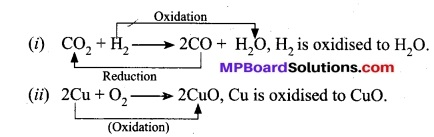(b) A reaction which involves loss of oxygen is called a reduction reaction.
For example:
(i) CuO + H2 → Cu + H2O, CuO is reduced to Cu.
(ii) ZnO + C → Zn + CO, ZnO is reduced to Zn.

Question 17.
A shiny brown coloured element ‘X’ on heating in the air becomes black in colour. Name the element ‘X’ and the black coloured compound formed.
‘X’ is copper (Cu) and the black coloured compound is a copper oxide (CuO). The equation of the reaction involved in heating copper is given below: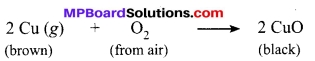Question 18.
Why do we apply paint on iron articles?
Iron articles are painted to prevent them from rusting. When painted, the contact of iron articles with atmospheric moisture and the air is cut off. Hence, rusting is prevented.

Question 19.
Oil and fat containing food items are flushed with nitrogen. Why?
Oil and fat containing food items are flushed with nitrogen to prevent the items from getting oxidised which may result in rancidity of such products. When fats and oils are oxidised, they become rancid and their smell and taste change. Nitrogen provides an inert atmosphere for them.

Question 20.
Explain the following terms with one example each.

1. Corrosion
2. Rancidity.

1. Corrosion:
When a metal is attacked by substances around it such as moisture, acids etc. it gets corroded and the process is called corrosion. For example, rusting of iron products.
2. Rancidity:
The Process in which fats and oils or food products made from fats or oils get oxidised resulting in a change of smell and the taste is called rancidity. For example, food items made from oil like chips becomes rancid if kept open for some time.

### MP Board Class 10th Science Chapter 1 Additional Questions

MP Board Class 10th Science Chapter 1 Multiple Choice Questions

Question 1.
The burning of magnesium in air results in weight gain of products, it shows:
(a) Physical change in magnesium
(b) A chemical reaction between magnesium and oxygen of air shift
(c) No change
(d) Both (a) and (b) are correct.
(b) A chemical reaction between magnesium and oxygen of air shift

Question 2.
Which one of the following is not a chemical reaction?
(a) Rusting of iron materials
(b) Cooking food
(c) Melting of ice
(d) Burning paper
(c) Melting of ice

Question 3.
Which one of the following is not a characteristic of a chemical change?
(a) Change in colour
(b) Evolution of gas
(c) Change in temperature
(d) Change in size
(d) Change in size

Question 4.
The balanced chemical reaction is represented with:
(a) The exact amount of material used.
(b) Correct formulation and their ratio being used.
(c) Products only.
(d) Reactants only.
(b) Correct formulation and their ratio being used.

Question 5.
Which one of the following is not mentioned in a balanced chemical reaction?
(a) Temperature and pressure
(b) State of reactants and products
(c) Mass of reactants and products
(d) Formulae of reactants and products
(b) State of reactants and products

Question 6.
The breaking down of food to CO2 and H2O is a type of:
(a) Exothermic reaction
(b) Endothermic reaction
(c) Reactions without temperature change
(d) Reactions without energy change
(a) Exothermic reaction

Question 7.
The burning of magnesium in the air is represented by the following equation:
2Mg + O2 → 2MgO
It is a type of:
(a) Displacement reaction
(b) Double displacement
(c) Combination reaction
(d) Corrosion
(c) Combination reaction

Question 8.
Which one of the following is a kind of combination reaction?
(a) 4Na(s) + O2(g) → 2Na2O(s)
(b) CuO(s)+ H2(g) → Cu(s) + H2O
(c) ZnO(s) + C(s) → Zn(s) + CO(g)
(d) None of above.
(a) 4Na(s) + O2(g) → 2Na2O(s)

Question 9.
Photosynthesis is a:
(i) Endothermic reaction
(ii) Exothermic reaction
(iii) Catalytic reaction
Choose correct combination:
(a) (i) and (ii)
(b) (i) and (iii)
(c) (ii) and (iii)
(d) (ii) only
(c) (ii) and (iii)

Question 10.
Dry fruits if not consumed for a long time get rotten because of:
(a) Temperature change
(b) Rusting
(c) Rancidity
(d) None of the above
(c) Rancidity

Question 11.
A chemical reaction involves:
(a) breaking of bonds
(b) formation of bonds
(c) no change
(d) both breaking and formation of bonds
(d) both breaking and formation of bonds

Question 12.
A balanced chemical equation has:
(a) Equality of mass at both side of reaction.
(b) Equality of atoms at both side of reaction.
(c) Equality of numbers of elements at both side of reaction.
(d) All of the above.
(d) All of the above.

Question 13.
Combustion of food at the cellular level of our body is:
(a) Oxidation
(b) Reduction
(c) Redox
(d) Heating
(a) Oxidation

Question 14.
Lemon contains:
(a) Formic acid
(b) Citric acid
(c) Succinic acid
(d) Ascorbic acid.
(b) Citric acid

Question 15.
Food gives a bad taste and a bad smell sometimes, because of:
(a) Rancidity
(b) Displacement
(c) Heating
(d) None
(a) Rancidity

Question 16.
The formation of water from its components gases is an example of:
(a) Combination reaction
(b) Oxidation reaction
(c) Decomposition reaction
(d) Reduction reaction
(a) Rancidity

Question 17.
(a) release of gas
(b) equilibrium
(c) formation of a precipitate
(d) lowering of temperature
(b) equilibrium

Question 18.
Silver develops a black colour layering after some time in the open air, because of:
(a) Rancidity
(b) Displacement
(c) Heating
(d) Corrosion
(d) Corrosion

MP Board Class 10th Science Chapter 1 Very Short Answer Type Questions

Question 1.
Is given reaction a balanced reaction?
2Mg + O2 → 2MgO.
Yes, it is.

Question 2.
Why gold and silver do not corrode?
It is because they are not very reactive.

Question 3.
Why does copper vessel acquire green coating in the open atmosphere?
It reacts with CO2 in the atmosphere and forms a layer of basic copper carbonate.

Question 4.
What type of reaction is the reaction between an acid and a base?
Neutralization reaction.

Question 5.
What type of reaction is rusting of iron?
Oxidation reaction.

Question 6.
What is the name of the gas which burns with a pop sound?
Hydrogen gas.

Question 7.
Why is hydrogen peroxide stored in coloured bottles?
To prevent photolytic decomposition of hydrogen peroxide, it is stored in coloured bottles.

Question 8.
Give two examples from everyday life situations where redox reactions are taking place.
Corrosion and rancidity.

Question 9.
In electrolysis of water, what is the volume of gas collected over electrode?
In water (H2O), hydrogen and oxygen are present in the ratio of 2:1 by volume.

Question 10.
What is the nature of change during a chemical reaction?
It is a permanent change.

Question 11.
What kind of change occurs during the dissolution of sugar in the water?
Physical change.

Question 12.
Give two examples of exothermic reactions.
Formation of ammonia and digestion of food.

Question 13.
Write three forms of energy evolution during the exothermic reaction.
Heat, sound and light evolution.

Question 14.
Where do we write or indicate the value of temperature used during a chemical reaction in a chemical equation?
Over the arrow or with the arrow.

Question 15.
What kind of chemical reaction occurs during the formation of nitrogen mono-oxide from nitrogen and oxygen gas?
Combination reaction.

Question 16.
Which catalyst is used in a given reaction?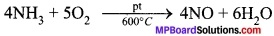Pt or platinum.

Question 17.
Give an example of a decomposition reaction.
Formation of calcium oxide from the decomposition of calcium carbonate,
CaCO3 $$\underrightarrow { \Delta }$$ CaO + CO2

Question 18.
What kind of chemical reaction occurs while rusting of silver?
Oxidation reaction.

MP Board Class 10th Science Chapter 1 Short Answer Type Questions

Question 1.
Write two important uses of the balancing equation.

1. It gives an idea of the ratio of reactants and products formed.
2. It verifies the law of conservation of mass.

Question 2.
Write two important checklists to analyse whether the equation is balanced or not.

1. Equal number of atoms on both sides.
2. Correct formulae of atoms and molecules on both sides.

Question 3.
Write two examples of each for physical and chemical change.
Physical change:

1. Melting of ice.
2. Evaporation.

Chemical change:

1. Cooking food.
2. Photosynthesis.

Question 4.
Balance the following equations:

1. HgO $$\underrightarrow { \Delta }$$ Hg + O2
2. KClO3 $$\underrightarrow { Mn{ O }_{ 2 } }$$ KCl + O2

Balanced equations are as follows:

1. 2HgO $$\underrightarrow { \Delta }$$ 2Hg + O2
2. 2 KClO3 $$\underrightarrow { Mn{ O }_{ 2 } }$$ 2KCl3 + 3O2

Question 5.
Name at least three types of chemical reactions with examples.

1. Combination reaction – Formation of water from gases.
2. Decomposition reaction – Digestion.
3. Oxidation reaction – Rusting.

Question 6.
What do you know about reversible reactions? Give an example of it.
Reactions in which products reform the reactant under suitable conditions are called reversible reaction.
Example:
Formation of ammonia: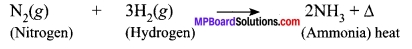Question 7.
Why gold plated articles over silver are available?
Gold is more reactive than silver, hence during electrodeposition, it replaces silver and gets deposited as a coating. During electroplating, displacement reaction occurs.

Question 8.
What do you mean by balanced chemical equation?
An equation that has an equal number of atoms of each element on both the sides of the equation is called the balanced chemical equation, i.e., the mass of the reactants is equal to the mass of the products.
e.g., 2Mg + O2 → 2MgO

Question 9.
Define rancidity.
When fats and oils are oxidized, they become rancid and their smell and taste change. This process is known as rancidity.

Question 10.
Give an example of a decomposition reaction where energy is supplied in the form of light.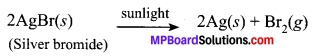Question 11.
Name the oxidizing agent and reducing agent in the following equation:
3MnO2(s) + 4Al(s) → 3Mn(s) + 2Al2O3(s)

1. Reducing agent: Al
2. Oxidizing agent: MnO2.

Question 12.
Explain how respiration is an exothermic reaction.
During digestion, food is broken down into simpler substances. For example, rice, potatoes and bread contain carbohydrates. These carbohydrates are broken down to form glucose. This glucose combines with oxygen in the cells of our body and provides energy. Hence, respiration is an exothermic process,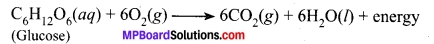Question 13.
Write factors influencing the rate of a chemical reaction.
Following are the factors that can influence the rate of reaction:

1. Nature of reactants (i.e., fast or slow).
2. The concentration of reactants.
3. Surface Area.
4. Temperature.
5. Catalyst.

Question 14.
Name one metal that when placed in ferrous sulphate solution will discharge its green colour.
Potassium (K):
‘K’ is more reactive than iron and will replace it. The green colour is due to the formation of a new compound.
2K + FeSO4 → K2SO4 + Fe

Question 15.
What is being added to the first reactant in reaction given below? (HOTS)

1. 2FeCl2 + Cl2 → 2FeCl3.
2. 2KI + H2O2 → I2 + 2KOH.

Question 16.
Which reactant is being oxidized in the following reactions? (HOTS)

1. 2Cu + O2 → 2CuO
2. H2S + Cl2 → 2HCl + S

1. ‘Cu’ is being oxidized.
2. ‘H’ is being oxidised.

MP Board Class 10th Science Chapter 1 Long Answer Type Questions

Question 1.
Write balanced chemical equations for the following reactions: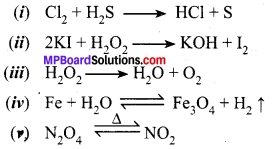Balanced chemical equations are: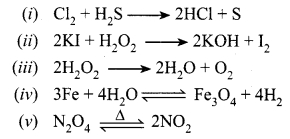Question 2.
Write balanced chemical equations for the following reactions and write the name of reactions and ratio of products formed for each of them.Balanced chemical equations are:Reactions and ratio of products:
(i) Redox or oxidation reaction ratio 2:1.
(ii) Oxidation reaction ratio 2:1.
(iii) Decomposition ratio 2:1.
(iv) Reversible and redox ratio 1 : 4.
(v) Decomposition ratio 2:1.

Question 3.
Rama bought a new set of an iron chair for her open garden space. After some months she found a dirty, brown coating over it. She painted the iron surface with colour to make it better for use. (Value-Based)

1. Why the chair gets brown, dirty?
2. Why did she use the paint?
3. What value does Rama show?

1. The outer surface which is made of iron reacts with weather’s moisture and gets rusted. Hence, gets the brown dirty coating.
2. Paint protects the outer surface of iron from getting rusted.
3. She protected the garden chair and showed her sense of awareness.

### MP Board Class 10th Science Chapter 1 Textbook Activities

Class 10 Science Activity 1.1 Page No. 1

Caution:

• This activity needs the teacher’s assistance. It would be better if students wear suitable eyeglasses.
• Clean a magnesium ribbon about 3-4 cm long by rubbing it with sandpaper.
• Hold it with a pair of tongs. Burn it using a spirit lamp or burner and collect the ash so formed in a watch-glass as shown in below Figure. Burn the magnesium ribbon keeping away as far as possible from your eyes.
• What do you observe?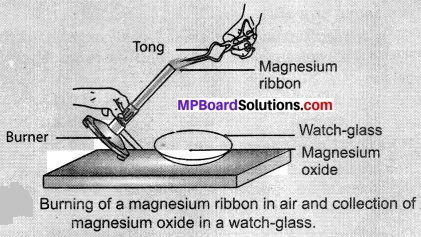Observations:
When we burn magnesium ribbon, it burns with crackling sound, dazzling light and produces ash. This ash contains oxide of magnesium,
2Mg(s) + O2(g) → 2MgO(s)

Class 10 Science Activity 1.2 Page No. 2

1. Take a lead nitrate solution in a test tube.
2. Add potassium iodide solution to this.
3. What do you observe?

Observations:
Lead iodide is formed when lead nitrate reacts with potassium iodide. Lead iodide is insoluble in water and yellow in colour.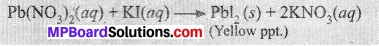Class 10 Science Activity 1.3 Page No. 2

1. Take a few zinc granules in a conical flask or a test tube.
2. Add dilute hydrochloric acid or sulphuric acid to this below figure.

Caution:

1. Handle the acid with care.
2. Do you observe anything happening around the zinc granules?
3. Touch the conical flask or test tube. Is there any change in its temperature?

Observations:
Zinc chloride is formed when zinc metal reacts with hydrochloric acid, bubbles of hydrogen gas are also observed in this process. The feat is evolved because it is an exothermic process.
Zn(s) + 2HCl(dil.) → ZnCl2(aq) + H2(g) + Δ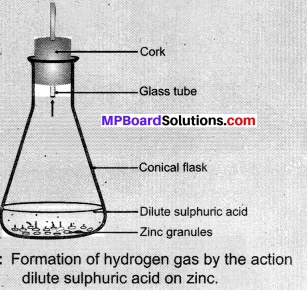Class 10 Science Activity 1.4 Page No. 6

1. Take a small amount of calcium oxide or quick lime in a busker.
2. Slowly add water to this.
3. Touch the beaker as shown in Figure.
4. Do you feel any change in temperature?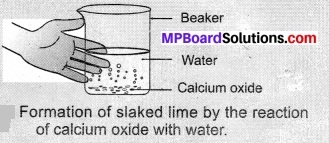Observations:
Slaked lime is produced when calcium oxide reacts with water, it is an exothermic reaction and produces a huge amount of energy as heat. It is a combination reaction,
CaO(s) + H2O(l) → Ca(OH)2(aq) + Δ

Class 10 Science Activity 1.5 Page No. 6

1. Take about 2 g ferrous sulphate crystals in a dry boiling tube.
2. Note the colour of the ferrous sulphate crystals.
3. Heat the boiling tube over the flame of a burner or spirit lamp as shown in Figure.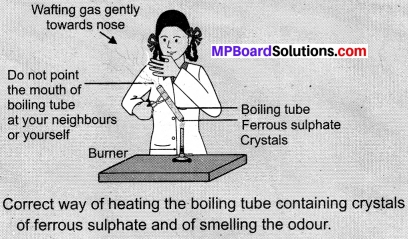Observations:
When we heat ferrous sulphate crystals in a boiling tube, a brownish-black ferric oxide is formed, SO2 and SO3 gas are escaped. Their presence is observed by the smell in the air. It is a decomposition reaction,
2FeSO4(s) → Fe2O3(s) + SO2(g) + SO3(g)

Class 10 Science Activity 1.6 Page No. 8

1. Take about 2 g lead nitrate powder in a boiling tube.
2. Hold the boiling tube with a pair of tongs and heat it over a flame. as shown in Figure.
3. What do you observe? Note down the change. If any.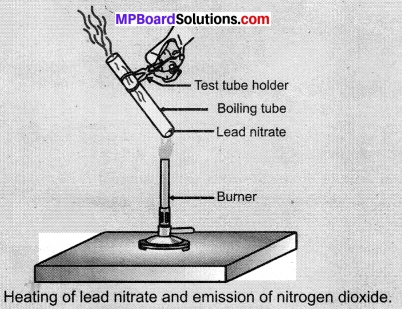Observations:
We will observe brown fumes emission from the test tube. Brown fumes signify nitrogen dioxide (NO2). It is a decomposition reaction.
2Pb(NO3)2(s) $$\underrightarrow { Heat }$$ 2PbO(s) + 4NO2(g) + O2(g)

Class 10 Science Activity 1.7 Page No. 8

1. Take a plastic mug. Drill two holes at its base and fit rubber stoppers in these holes. Insert carbon electrodes in these rubber stoppers as shown in Figure.
2. Connect these electrodes to a 6-volt battery.
3. Fill the mug with water such that the electrodes are immersed. Add a few drops of dilute sulphuric acid to the water.
4. Take two test tubes filled with water and invert them over the two carbon electrodes.
5. Switch on the current and leave the apparatus undisturbed for some time.
6. You will observe the formation of bubbles at both electrodes. These bubbles displace water in the test tubes.
7. Is the volume of the gas collected the same in both the test tubes?
8. Once the test tubes are filled with the respective gases. Remove them carefully.
9. Test these gases one by one by bringing a burning candle close to the mouth of the test tubes.

Caution:

1. This step must be performed carefully by the teacher.
2. What happens in each case?
3. Which gas is present in each test tube?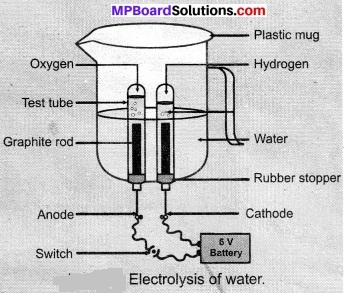Observations:
On electrolysis of water, hydrogen and oxygen gas is produced. Hydrogen gas has twice the volume of oxygen gas. Oxygen gas let the burning continue while hydrogen gas burns with a pop sound. The gas collected at the cathode is oxygen while gas collected at the anode is hydrogen.

Class 10 Science Activity 1.8 Page No. 9

1. Take about 2 g silver chloride in a china dish.
2. What is its colour?
3. Place this china dish in sunlight for some time Figure.
4. Observe the colour of the silver chloride after some time.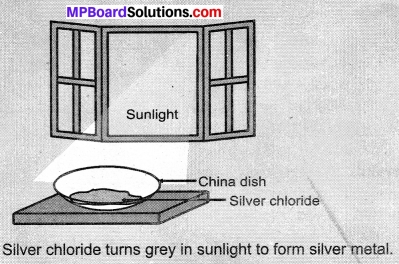Observations:
White silver chloride turns grey in sunlight due to the decomposition of silver chloride into silver and chlorine gas by light
2AgCl(s) $$\underrightarrow { Sunlight }$$ 2Ag(s) + Cl2(g)

Class 10 Science Activity 1.9 Page No. 10

1. Take three iron nails and clean them by rubbing with sandpaper.
2. Take two test tubes marked as (A) and (B). In each test tube, take about 10mL copper sulphate solution.
3. Tie two iron nails with a thread and immerse them carefully in the copper sulphate solution in test tube B for about 20 minutes [Fig. 1.8(a)]. Keep one iron nail aside for comparison.
4. After 20 minutes, take out the iron nails from the copper sulphate solution.
5. Compare the intensity of the blue colour of copper sulphate solutions in test tubes (A) and (B), [Fig. 1.8 (b)].
6. Also, compare the colour of the iron nails dipped in the copper sulphate solution with the one kept aside [Fig. 1.8 (b)].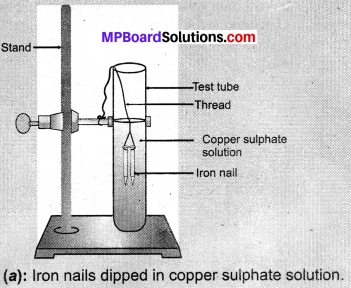Observations:

1. When we dip the iron nail in copper sulphate solution its blue colour changes due to the formation of iron sulphate and represents displacement reaction.
Fe(s) + CuSO4 (aq) → FeSO4(aq) + Cu(s)
2. Copper sulphate in test tube A is dark blue and its colour in test tube B is light blue.
3. An iron nail becomes brownish when we put them in test tube B because of Cu deposition over it and solution turns green due to formation of FeSO4.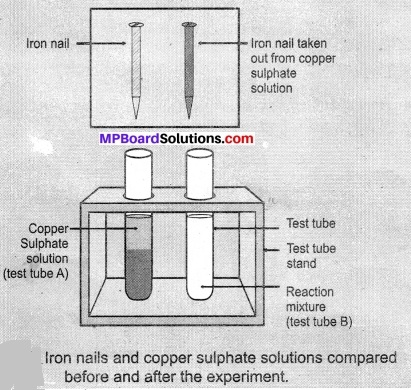Class 10 Science Activity 1.10 Page No. 10

1. Take about 3 ml of sodium sulphate solution in a test tube.
2. In another test tube, take about 3 ml of barium chloride solution.
3. Mix the two solutions
4. What do you observe?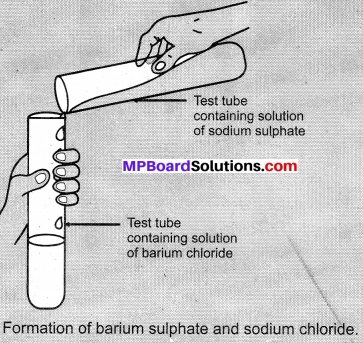Observations:
We get a white precipitate of BaSO4 when sodium sulphate reacts with barium chloride.
Na2SO4(aq) + BaCl2(aq) → BaSO4(s) + 2NaCl

Class 10 Science Activity 1.11 Page No. 12

1. Heat a china dish containing about 1 g copper powder Figure.
2. What do you observe?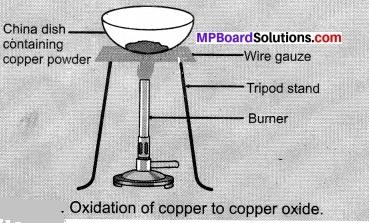Observations:
Black colour copper oxide is formed.
2Cu + O2 $$\underrightarrow { \Delta }$$ 2CuO

We hope the given MP Board Class 10th Science Solutions Chapter 1 Chemical Reactions and Equations will help you. If you have any queries regarding NCERT Madhya Pradesh Syllabus MP Board Class 10 Science Book Solutions Chapter 1 Chemical Reactions and Equations, drop a comment below and we will get back to you at the earliest.